## 1、创建组的+号位置

WPS和高版本OFFICE版本设置方式：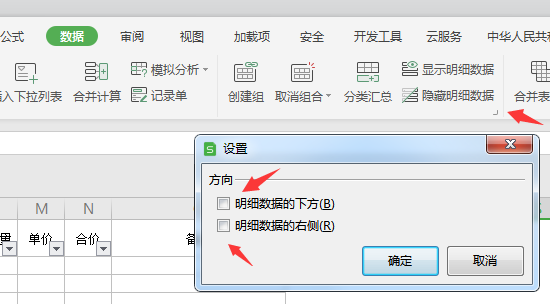## 2、提取单元格中的数字``  =LEFT(A2,3)``

LEFT函数表示从左边提取数字，并返回指定个数的字符。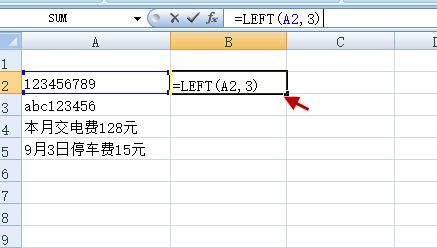``  =MID(A3,4,6)``

MID函数表示从指定位置返回指定长度的字符。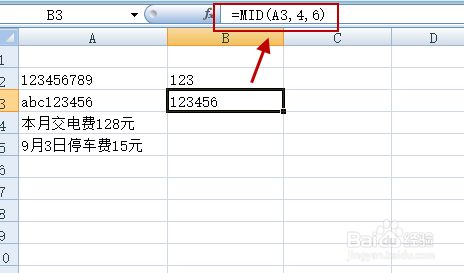``  =MID(A4,6,3)``

MID函数表示从指定位置返回指定长度的字符。注意：一个中文字符仍只算1位的。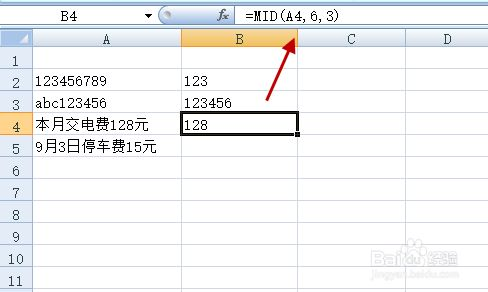`` =LEFT(A4,LEN(A4)-LEN("元"))``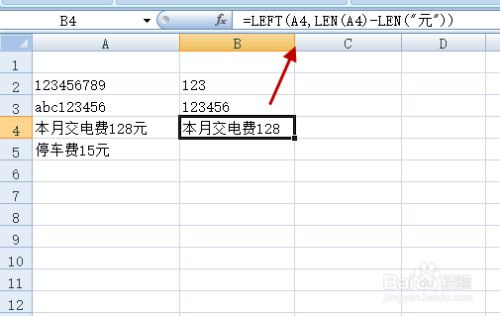`` =RIGHT(B4,2*LEN(B4)-LENB(B4))``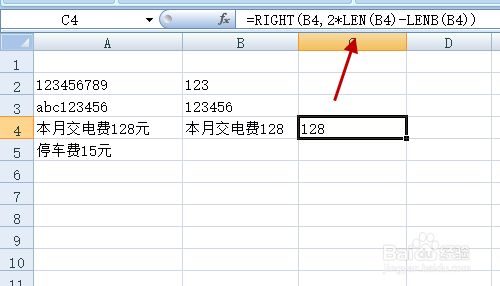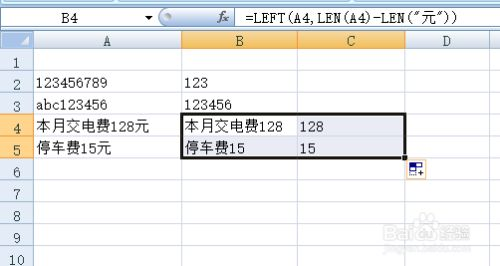Last modification：February 16th, 2022 at 06:42 pm